##ifWithoutElse### Use

If the if-condition of ifWithoutElse gets the value true, the input value of then is returned as the result. In this way, you can use this function as a filter in target-field mappings.

### What Does the Function Return as the Else Value?

To investigate the different cases in which the ifcondition does not get the value true, we will use the following outbound message:

 Outbound Message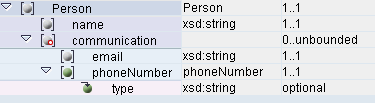In this structure, the e-mail addresses and telephone numbers of one person (root element Person) can be created within a single element communication that occurs multiple times. The element phoneNumber from the outbound message can have different types. They are saved as an enumeration for the attribute type of the element. The enumeration has the values private, office, and fax. A test instance for the given outbound message might look as follows:

 Test Instance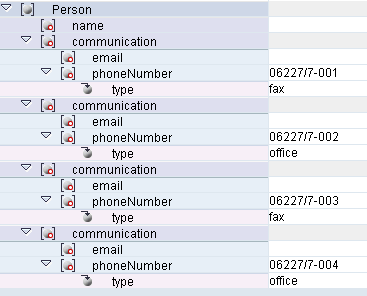The Else value of ifWithoutElse depends on whether the result of this function is transferred to a target field or to a function. We will now look at both cases by using the outbound message and test instance above. In both cases, only the fax numbers are to be transferred to a target field faxNumber.

### Else Value for Target Fields

In the target-field mapping in the table below, the result value of ifWithoutElse is transferred directly to the target field faxNumber.

 Target-Field Mapping Queue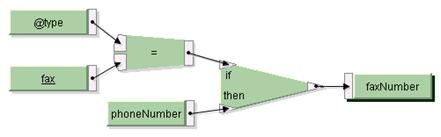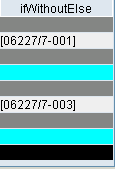In such cases, you want to either create the target element faxNumber and use phoneNumber as the fax number, or the target element is not to be created at all. Correspondingly, ifWithoutElse creates the value ResultList.SUPPRESS if the if-condition is not satisfied. The second column in the table displays the queue for ifWithoutElse in this target-field mapping with the test instance above: whenever type is not equal to “fax“, ResultList.SUPPRESS is shown in the queue.

### Else Value for Functions

Transferring the result value of ifWithoutElse to a standard function with multiple input parameters can have two different effects:

ifWithoutElse acts like a filter. If you only require as input parameters those values in the subsequent function for which the if-condition was satisfied when filtering, choose the empty string as the Else value for ifWithoutElse. In this case, the standard function does not interpret the entry as a value and skips it.

If the input queues in the standard function are to be synchronized, ifWithoutElse must get the value ResultList.SUPPRESS. In this case, the standard function does not skip the SUPPRESS value.

#### Example: Empty Value as Else Value

In the following target-field mapping, the result value of ifWithoutElse is transferred to the standard function concat. The aim is to number all the telephone numbers that refer to a fax. We will therefore use the counter and concatfunctions with a blank as a divider: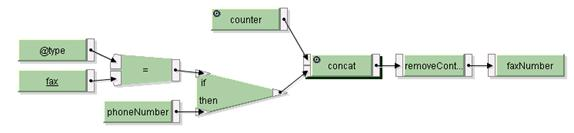For each communication element there is only one telephone number or e-mail address. Thus, after each number the context changes. You can specify whether the Else value is an empty string or the SUPPRESS value by using the function parameters of ifWithouElse. The following table shows the different result queues of concat, depending on how the function parameters of ifWithoutElse are set:

 No SUPPRESS Value as Else Value SUPPRESS Value as Else Value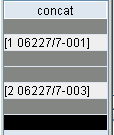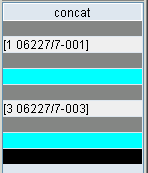The numbering is different because the standard function concat skips the context with the empty string while the SUPPRESS value is linked to the second value of counter.

#### SUPPRESS Value as Else Value

As you can see in the last example, using the SUPPRESS value ensures that no contexts are skipped when the result is calculated by the standard function. This is necessary, for example, if instead of the counterfunction used above, an output field name from another context such as phoneNumber gets the input parameter, for which there are the same number of values per context. In this case, by using the SUPPRESS value as the Else value, both inbound queues have the same number of values per context.